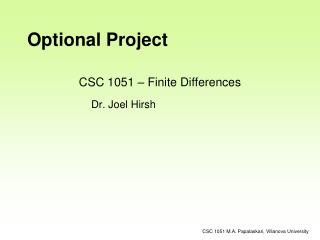Download PresentationCSC 1051 – Finite Differences# CSC 1051 – Finite Differences - PowerPoint PPT Presentation

Download Presentation##### CSC 1051 – Finite Differences

Download Policy: Content on the Website is provided to you AS IS for your information and personal use and may not be sold / licensed / shared on other websites without getting consent from its author. While downloading, if for some reason you are not able to download a presentation, the publisher may have deleted the file from their server.

- - - - - - - - - - - - - - - - - - - - - - - - - - - E N D - - - - - - - - - - - - - - - - - - - - - - - - - - -
##### Presentation Transcript

1. CSC 1051 – Finite Differences Optional Project Dr. Joel Hirsh CSC 1051 M.A. Papalaskari, Villanova University

2. Description • Derivatives or slopes of a function are used in many numerical simulations: • Numerical weather prediction • Aircraft design • Financial modeling. • These derivatives need to be computed using some type of approximation. One of the most commonly used approximations is the finite difference. There are many types of finite difference approximations. In this project, we will use two of the simplest: • Forward differences • Central differences. CSC 1051 M.A. Papalaskari, Villanova University

3. Definitions • Given a function of a single variable, x, let f(x) be some single valued function of x, e.g., the trigonometric function sin(x). Let the derivative of f(x) be f’(x). For some small interval, h, • The forward difference approximation is • The central difference approximation is CSC 1051 M.A. Papalaskari, Villanova University

4. Errors • If f’(x) is well known then the error in the two methods can be expressed as • efd(x) = fd(x) – f’(x) = O(h) • ecd(x) = cd(x) – f’(x) = O(h2) • Where O(y) means that the quantity goes to zero in limit as y goes to zero. CSC 1051 M.A. Papalaskari, Villanova University

5. Assignment • Write a Java program using the function f(x) = sin(x). Input a value of x. Evaluate the function, and both types of finite difference methods over a range of values for h, start with h = 0.1 and half the value of h 8 times. For each values of h, print the function value, the exact derivative (the exact derivative of sin(x) is cos(x)), the two finite differences and the two errors. Your output should be a table and look like CSC 1051 M.A. Papalaskari, Villanova University

6. Sample Output CSC 1051 M.A. Papalaskari, Villanova University

7. Excel Plot of Sample Output CSC 1051 M.A. Papalaskari, Villanova University